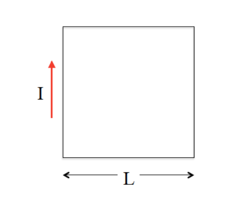# Problem: A conductor in the shape of a square loop of edge length L carries a current I as shown in the figure below. Calculate the magnitude and direction of the magnetic field at the center of the square.

###### FREE Expert Solution
97% (469 ratings)
###### Problem Details

A conductor in the shape of a square loop of edge length L carries a current I as shown in the figure below. Calculate the magnitude and direction of the magnetic field at the center of the square.Frequently Asked Questions

What scientific concept do you need to know in order to solve this problem?

Our tutors have indicated that to solve this problem you will need to apply the Biot-Savart Law (Calculus) concept. You can view video lessons to learn Biot-Savart Law (Calculus). Or if you need more Biot-Savart Law (Calculus) practice, you can also practice Biot-Savart Law (Calculus) practice problems.

How long does this problem take to solve?

Our expert Physics tutor, Douglas took 21 minutes and 12 seconds to solve this problem. You can follow their steps in the video explanation above.

What professor is this problem relevant for?

Based on our data, we think this problem is relevant for Professor Deegan's class at UMICH.